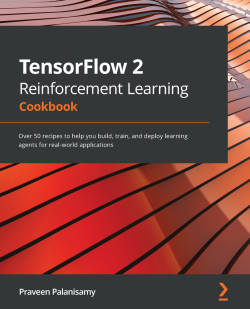# TensorFlow 2 Reinforcement Learning Cookbook

By Praveen Palanisamy
• \$33.99 eBook
• \$46.99 Print + eBook
• \$12.99 eBook + Subscription
What do you get with a Packt Subscription?

• Constantly updated with 100+ new titles each month
• Breadth and depth in over 1,000+ technologies

With deep reinforcement learning, you can build intelligent agents, products, and services that can go beyond computer vision or perception to perform actions. TensorFlow 2.x is the latest major release of the most popular deep learning framework used to develop and train deep neural networks (DNNs). This book contains easy-to-follow recipes for leveraging TensorFlow 2.x to develop artificial intelligence applications.

Starting with an introduction to the fundamentals of deep reinforcement learning and TensorFlow 2.x, the book covers OpenAI Gym, model-based RL, model-free RL, and how to develop basic agents. You'll discover how to implement advanced deep reinforcement learning algorithms such as actor-critic, deep deterministic policy gradients, deep-Q networks, proximal policy optimization, and deep recurrent Q-networks for training your RL agents. As you advance, you’ll explore the applications of reinforcement learning by building cryptocurrency trading agents, stock/share trading agents, and intelligent agents for automating task completion. Finally, you'll find out how to deploy deep reinforcement learning agents to the cloud and build cross-platform apps using TensorFlow 2.x.

By the end of this TensorFlow book, you'll have gained a solid understanding of deep reinforcement learning algorithms and their implementations from scratch.

Publication date:
January 2021
Publisher
Packt
Pages
472
ISBN
9781838982546

# Chapter 1: Developing Building Blocks for Deep Reinforcement Learning Using Tensorflow 2.x

This chapter provides a practical and concrete description of the fundamentals of Deep Reinforcement Learning (Deep RL) filled with recipes for implementing the building blocks using the latest major version of TensorFlow 2.x. It includes recipes for getting started with RL environments, OpenAI Gym, developing neural network-based agents, and evolutionary neural agents for addressing applications with both discrete and continuous value spaces for Deep RL.

The following recipes are discussed in this chapter:

• Building an environment and reward mechanism for training RL agents
• Implementing neural network-based RL policies for discrete action spaces and decision-making problems
• Implementing neural network-based RL policies for continuous action spaces and continuous-control problems
• Working with OpenAI Gym for RL training environments
• Building a neural agent
• Building a neural evolutionary agent

# Technical requirements

The code in the book has been extensively tested on Ubuntu 18.04 and Ubuntu 20.04 and should work with later versions of Ubuntu as long as Python 3.6+ is available. With Python 3.6 installed along with the necessary Python packages as listed before the start of each of the recipes, the code should run fine on Windows and macOS X too. It is advised to create and use a Python virtual environment named `tf2rl-cookbook` to install the packages and run the code in this book. Miniconda or Anaconda installation for Python virtual environment management is recommended.The complete code for each recipe in this chapter will be available here: https://github.com/PacktPublishing/Tensorflow-2-Reinforcement-Learning-Cookbook.

# Building an environment and reward mechanism for training RL agents

This recipe will walk you through the steps to build a Gridworld learning environment to train RL agents. Gridworld is a simple environment where the world is represented as a grid. Each location on the grid can be referred to as a cell. The goal of an agent in this environment is to find its way to the goal state in a grid like the one shown here: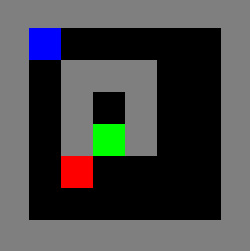Figure 1.1 – A screenshot of the Gridworld environment

The agent's location is represented by the blue cell in the grid, while the goal and a mine/bomb/obstacle's location is represented in the grid using green and red cells, respectively. The agent (blue cell) needs to find its way through the grid to reach the goal (green cell) without running over the mine/bomb (red cell).

To complete this recipe, you will first need to activate the `tf2rl-cookbook` Python/Conda virtual environment and `pip install numpy gym`. If the following import statements run without issues, you are ready to get started!

```import copy
import sys
import gym
import numpy as np```

Now we can begin.

## How to do it…

To train RL agents, we need a learning environment that is akin to the datasets used in supervised learning. The learning environment is a simulator that provides the observation for the RL agent, supports a set of actions that the RL agent can perform by executing the actions, and returns the resultant/new observation as a result of the agent taking the action.

Perform the following steps to implement a Gridworld learning environment that represents a simple 2D map with colored cells representing the location of the agent, goal, mine/bomb/obstacle, wall, and empty space on a grid:

1. We'll start by first defining the mapping between different cell states and their color codes to be used in the Gridworld environment:
```EMPTY = BLACK = 0
WALL = GRAY = 1
AGENT = BLUE = 2
MINE = RED = 3
GOAL = GREEN = 4
SUCCESS = PINK = 5```
2. Next, generate a color map using RGB intensity values:
```COLOR_MAP = {
BLACK: [0.0, 0.0, 0.0],
GRAY: [0.5, 0.5, 0.5],
BLUE: [0.0, 0.0, 1.0],
RED: [1.0, 0.0, 0.0],
GREEN: [0.0, 1.0, 0.0],
PINK: [1.0, 0.0, 1.0],
}```
3. Let's now define the action mapping:
```NOOP = 0
DOWN = 1
UP = 2
LEFT = 3
RIGHT = 4```
4. Let's then create a `GridworldEnv` class with an `__init__` function to define necessary class variables, including the observation and action space:
```class GridworldEnv():
def __init__(self):```

We will implement `__init__()` in the following steps.

5. In this step, let's define the layout of the Gridworld environment using the grid cell state mapping:
```	self.grid_layout = """
1 1 1 1 1 1 1 1
1 2 0 0 0 0 0 1
1 0 1 1 1 0 0 1
1 0 1 0 1 0 0 1
1 0 1 4 1 0 0 1
1 0 3 0 0 0 0 1
1 0 0 0 0 0 0 1
1 1 1 1 1 1 1 1
"""```

In the preceding layout, `0` corresponds to the empty cells, `1` corresponds to walls, `2` corresponds to the agent's starting location, `3` corresponds to the location of the mine/bomb/obstacle, and `4` corresponds to the goal location based on the mapping we defined in step 1.

6. Now, we are ready to define the observation space for the Gridworld RL environment:
```	self.initial_grid_state = np.fromstring(
self.grid_layout, dtype=int, sep=" ")
self.initial_grid_state = \
self.initial_grid_state.reshape(8, 8)
self.grid_state = copy.deepcopy(
self.initial_grid_state)
self.observation_space = gym.spaces.Box(
low=0, high=6, shape=self.grid_state.shape
)
self.img_shape = [256, 256, 3]
7. Let's define the action space and the mapping between the actions and the movement of the agent in the grid:
```	   self.action_space = gym.spaces.Discrete(5)
self.actions = [NOOP, UP, DOWN, LEFT, RIGHT]
self.action_pos_dict = {
NOOP: [0, 0],
UP: [-1, 0],
DOWN: [1, 0],
LEFT: [0, -1],
RIGHT: [0, 1],
}```
8. Let's now wrap up the `__init__` function by initializing the agent's start and goal states using the `get_state()` method (which we will implement in the next step):
```(self.agent_start_state, self.agent_goal_state,) = \
self.get_state()```
9. Now we need to implement the `get_state()` method that returns the start and goal state for the Gridworld environment:
```def get_state(self):
start_state = np.where(self.grid_state == AGENT)
goal_state = np.where(self.grid_state == GOAL)
start_or_goal_not_found = not (start_state \
and goal_state)
if start_or_goal_not_found:
sys.exit(
"Start and/or Goal state not present in
the Gridworld. "
"Check the Grid layout"
)
start_state = (start_state,
start_state)
goal_state = (goal_state, goal_state)
return start_state, goal_state```
10. In this step, we will be implementing the `step(action)` method to execute the action and retrieve the next state/observation, the associated reward, and whether the episode ended:
```def step(self, action):
"""return next observation, reward, done, info"""
action = int(action)
info = {"success": True}
done = False
reward = 0.0
next_obs = (
self.agent_state + \
self.action_pos_dict[action],
self.agent_state + \
self.action_pos_dict[action],
)```
11. Next, let's specify the rewards and finally, return `grid_state`, `reward`, `done`, and `info`:
``` # Determine the reward
if action == NOOP:
return self.grid_state, reward, False, info
next_state_valid = (
next_obs < 0 or next_obs >= \
self.grid_state.shape
) or (next_obs < 0 or next_obs >= \
self.grid_state.shape)
if next_state_valid:
info["success"] = False
return self.grid_state, reward, False, info
next_state = self.grid_state[next_obs,
next_obs]
if next_state == EMPTY:
self.grid_state[next_obs,
next_obs] = AGENT
elif next_state == WALL:
info["success"] = False
reward = -0.1
return self.grid_state, reward, False, info
elif next_state == GOAL:
done = True
reward = 1
elif next_state == MINE:
done = True
reward = -1        # self._render("human")
self.grid_state[self.agent_state,
self.agent_state] = EMPTY
self.agent_state = copy.deepcopy(next_obs)
return self.grid_state, reward, done, info```
12. Up next is the `reset()` method, which resets the Gridworld environment when an episode completes (or if a request to reset the environment is made):
```def reset(self):
self.grid_state = copy.deepcopy(
self.initial_grid_state)
(self.agent_state, self.agent_goal_state,) = \
self.get_state()
return self.grid_state```
13. To visualize the state of the Gridworld environment in a human-friendly manner, let's implement a render function that will convert the `grid_layout` that we defined in step 5 to an image and display it. With that, the Gridworld environment implementation will be complete!
```def gridarray_to_image(self, img_shape=None):
if img_shape is None:
img_shape = self.img_shape
observation = np.random.randn(*img_shape) * 0.0
scale_x = int(observation.shape / self.grid_\
state.shape)
scale_y = int(observation.shape / self.grid_\
state.shape)
for i in range(self.grid_state.shape):
for j in range(self.grid_state.shape):
for k in range(3):  # 3-channel RGB image
pixel_value = \
COLOR_MAP[self.grid_state[i, j]][k]
observation[
i * scale_x : (i + 1) * scale_x,
j * scale_y : (j + 1) * scale_y,
k,
] = pixel_value
return (255 * observation).astype(np.uint8)
def render(self, mode="human", close=False):
if close:
if self.viewer is not None:
self.viewer.close()
self.viewer = None
return
img = self.gridarray_to_image()
if mode == "rgb_array":
return img
elif mode == "human":
from gym.envs.classic_control import \
rendering
if self.viewer is None:
self.viewer = \
rendering.SimpleImageViewer()
self.viewer.imshow(img)```
14. To test whether the environment is working as expected, let's add a `__main__` function that gets executed if the environment script is run directly:
```if __name__ == "__main__":
env = GridworldEnv()
obs = env.reset()
# Sample a random action from the action space
action = env.action_space.sample()
next_obs, reward, done, info = env.step(action)
print(f"reward:{reward} done:{done} info:{info}")
env.render()
env.close()```
15. All set! The Gridworld environment is ready and we can quickly test it by running the script (`python envs/gridworld.py`). An output such as the following will be displayed:
`reward:0.0 done:False info:{'success': True}`

The following rendering of the Gridworld environment will also be displayed: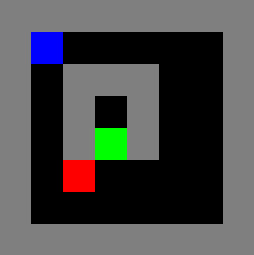Figure 1.2 – The Gridworld

Let's now see how it works!

## How it works…

The `grid_layout` defined in step 5 in the How to do it… section represents the state of the learning environment. The Gridworld environment defines the observation space, action spaces, and the rewarding mechanism to implement a Markov Decision Process (MDP). We sample a valid action from the action space of the environment and step the environment with the chosen action, which results in the new observation, reward, and a done status Boolean (representing if the episode has finished) as the response from the Gridworld environment. The `env.render()` method converts the environment's internal grid representation to an image and displays it for visual understanding.

# Implementing neural network-based RL policies for discrete action spaces and decision-making problems

Many environments (both simulated and real) for RL requires the RL agent to choose an action from a list of actions or, in other words, take discrete actions. While simple linear functions can be used to represent policies for such agents, they are often not scalable to complex problems. A non-linear function approximator such as a (deep) neural network can approximate arbitrary functions, even those required to solve complex problems.

The neural network-based policy network is a crucial building block for advanced RL and Deep RL and will be applicable to general, discrete decision-making problems.

By the end of this recipe, you will have an agent with a neural network-based policy implemented in TensorFlow 2.x that can take actions in the Gridworld environment and (with little or no modifications) in any discrete-action space environment.

Activate the `tf2rl-cookbook` Python virtual environment and run the following to install and import the packages:

```pip install --upgrade numpy tensorflow tensorflow_probability seaborn
import seaborn as sns
import tensorflow as tf
from tensorflow import keras
from tensorflow.keras import layers
import tensorflow_probability as tfp```

Let's get started.

## How to do it…

We will look at policy distribution types that can be used by agents in environments with discrete action spaces:

1. Let's begin by creating a binary policy distribution in TensorFlow 2.x using the `tensorflow_probability` library:
```binary_policy = tfp.distributions.Bernoulli(probs=0.5)
for i in range(5):
action = binary_policy.sample(1)
print("Action:", action)```

The preceding code should print something like the following:

```Action: tf.Tensor(, shape=(1,), dtype=int32)
Action: tf.Tensor(, shape=(1,), dtype=int32)
Action: tf.Tensor(, shape=(1,), dtype=int32)
Action: tf.Tensor(, shape=(1,), dtype=int32)
Action: tf.Tensor(, shape=(1,), dtype=int32)```

Important note

The values of the action that you get will differ from what is shown here because they will be sampled from the Bernoulli distribution, which is not a deterministic process.

2. Let's quickly visualize the binary policy distribution:
```# Sample 500 actions from the binary policy distribution
sample_actions = binary_policy.sample(500)
sns.distplot(sample_actions)```

The preceding code will generate a distribution plot as shown here: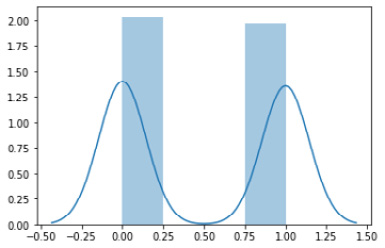Figure 1.3 – A distribution plot of the binary policy

3. In this step, we will be implementing a discrete policy distribution. A categorical distribution over a single discrete variable with k finite categories is referred to as a multinoulli distribution. The generalization of the multinoulli distribution to multiple trials is the multinomial distribution that we will be using to represent discrete policy distributions:
```action_dim = 4  # Dimension of the discrete action space
action_probabilities = [0.25, 0.25, 0.25, 0.25]
discrete_policy = tfp.distributions.Multinomial(probs=action_probabilities, total_count=1)
for i in range(5):
action = discrete_policy.sample(1)
print(action)```

The preceding code should print something along the lines of the following:

Important note

The values of the action that you get will differ from what is shown here because they will be sampled from the multinomial distribution, which is not a deterministic process.

```tf.Tensor([[0. 0. 0. 1.]], shape=(1, 4), dtype=float32)
tf.Tensor([[0. 0. 1. 0.]], shape=(1, 4), dtype=float32)
tf.Tensor([[0. 0. 1. 0.]], shape=(1, 4), dtype=float32)
tf.Tensor([[1. 0. 0. 0.]], shape=(1, 4), dtype=float32)
tf.Tensor([[0. 1. 0. 0.]], shape=(1, 4), dtype=float32)```
4. Next, we visualize the discrete probability distribution:
`sns.distplot(discrete_policy.sample(1))`

The preceding code will generate a distribution plot, like the one shown here for `discrete_policy`: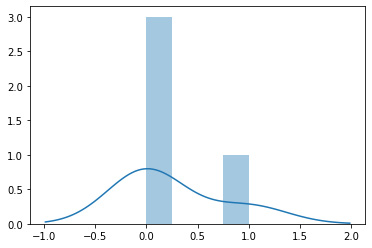Figure 1.4 – A distribution plot of the discrete policy

5. Then, calculate the entropy of a discrete policy:
```def entropy(action_probs):
return -tf.reduce_sum(action_probs * \
tf.math.log(action_probs), axis=-1)
action_probabilities = [0.25, 0.25, 0.25, 0.25]
print(entropy(action_probabilities))```
6. Also, implement a discrete policy class:
```class DiscretePolicy(object):
def __init__(self, num_actions):
self.action_dim = num_actions
def sample(self, actino_logits):
self.distribution = tfp.distributions.Multinomial(logits=action_logits, total_count=1)
return self.distribution.sample(1)
def get_action(self, action_logits):
action = self.sample(action_logits)
return np.where(action)[-1]
# Return the action index
def entropy(self, action_probabilities):
return – tf.reduce_sum(action_probabilities * tf.math.log(action_probabilities), axis=-1)```
7. Now we implement a helper method to evaluate the agent in a given environment:
```def evaluate(agent, env, render=True):
obs, episode_reward, done, step_num = env.reset(),
0.0, False, 0
while not done:
action = agent.get_action(obs)
obs, reward, done, info = env.step(action)
episode_reward += reward
step_num += 1
if render:
env.render()
return step_num, episode_reward, done, info```
8. Let's now implement a neural network Brain class using TensorFlow 2.x:
```class Brain(keras.Model):
def __init__(self, action_dim=5,
input_shape=(1, 8 * 8)):
"""Initialize the Agent's Brain model
Args:
action_dim (int): Number of actions
"""
super(Brain, self).__init__()
self.dense1 = layers.Dense(32, input_shape=\
input_shape, activation="relu")
self.logits = layers.Dense(action_dim)
def call(self, inputs):
x = tf.convert_to_tensor(inputs)
if len(x.shape) >= 2 and x.shape != 1:
x = tf.reshape(x, (1, -1))
return self.logits(self.dense1(x))
def process(self, observations):
# Process batch observations using `call(inputs)` behind-the-scenes
action_logits = \
self.predict_on_batch(observations)
return action_logits```
9. Let's now implement a simple agent class that uses a `DiscretePolicy` object to act in discrete environments:
```class Agent(object):
def __init__(self, action_dim=5,
input_dim=(1, 8 * 8)):
self.brain = Brain(action_dim, input_dim)
self.policy = DiscretePolicy(action_dim)
def get_action(self, obs):
action_logits = self.brain.process(obs)
action = self.policy.get_action(
np.squeeze(action_logits, 0))
return action```
10. Let's now test the agent in `GridworldEnv`:
```from envs.gridworld import GridworldEnv
env = GridworldEnv()
agent = Agent(env.action_space.n,
env.observation_space.shape)
steps, reward, done, info = evaluate(agent, env)
print(f"steps:{steps} reward:{reward} done:{done} info:{info}")
env.close()```

This shows how to implement the policy. We will see how this works in the following section.

## How it works…

One of the central components of an RL agent is the policy function that maps between observations and actions. Formally, a policy is a distribution over actions that prescribes the probabilities of choosing an action given an observation.

In environments where the agent can take at most two different actions, for example, in a binary action space, we can represent the policy using a Bernoulli distribution, where the probability of taking action 0 is given by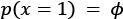, and the probability of taking action 1 is given by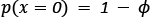, which gives rise to the following probability distribution: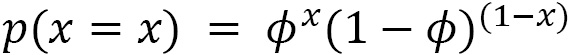A discrete probability distribution can be used to represent an RL agent's policy when the agent can take one of k possible actions in an environment.

In a general sense, such distributions can be used to describe the possible results of a random variable that can take one of k possible categories and is therefore also called a categorical distribution. This is a generalization of the Bernoulli distribution to k-way events and is therefore a multinoulli distribution.

# Implementing neural network-based RL policies for continuous action spaces and continuous-control problems

Reinforcement learning has been used to achieve the state of the art in many control problems, not only in games as varied as Atari, Go, Chess, Shogi, and StarCraft, but also in real-world deployments, such as HVAC control systems.

In environments where the action space is continuous, meaning that the actions are real-valued, a real-valued, continuous policy distribution is necessary. A continuous probability distribution can be used to represent an RL agent's policy when the action space of the environment contains real numbers. In a general sense, such distributions can be used to describe the possible results of a random variable when the random variable can take any (real) value.

Once the recipe is complete, you will have a complete script to control a car in two dimensions to drive up a hill using the `MountainCarContinuous` environment with a continuous action space. A screenshot from the `MountainCarContinuous` environment is shown here: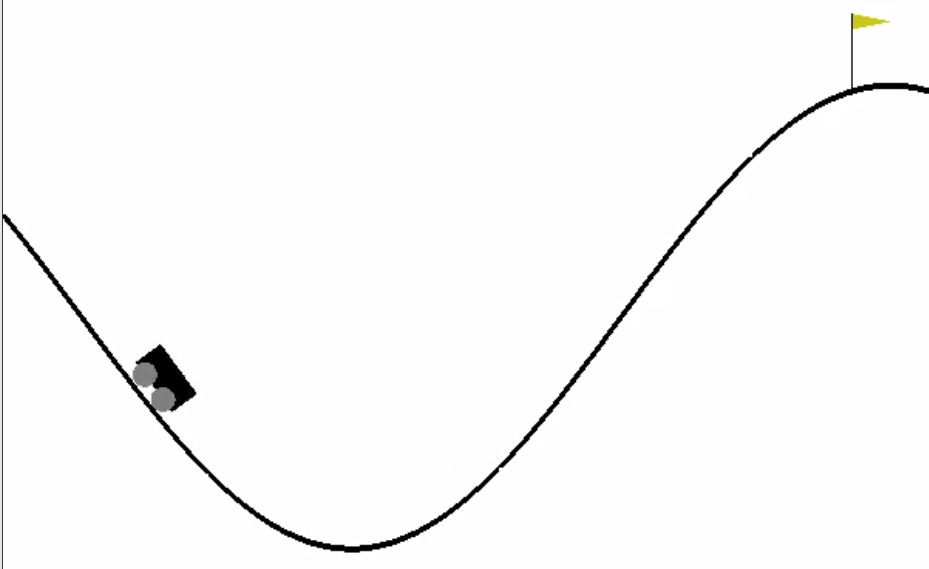Figure 1.5 – A screenshot of the MountainCarContinuous environment

Activate the `tf2rl-cookbook` Conda Python environment and run the following command to install and import the necessary Python packages for this recipe:

```pip install --upgrade tensorflow_probability
import tensorflow_probability as tfp
import seaborn as sns```

Let's get started.

## How to do it…

We will begin by creating continuous policy distributions using TensorFlow 2.x and the `tensorflow_probability` library and build upon the necessary action sampling methods to generate action for a given continuous space of an RL environment:

1. We create a continuous policy distribution in TensorFlow 2.x using the `tensorflow_probability` library. We will use a Gaussian/normal distribution to create a policy distribution over continuous values:
```sample_actions = continuous_policy.sample(500)
sns.distplot(sample_actions)```
2. Next, we visualize a continuous policy distribution:
```sample_actions = continuous_policy.sample(500)
sns.distplot(sample_actions)```

The preceding code will generate a distribution plot of the continuous policy, like the plot shown here: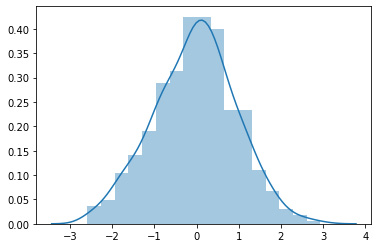Figure 1.6 – A distribution plot of the continuous policy

3. Let's now implement a continuous policy distribution using a Gaussian/normal distribution:
```mu = 0.0  # Mean = 0.0
sigma = 1.0  # Std deviation = 1.0
continuous_policy = tfp.distributions.Normal(loc=mu,
scale=sigma)
# action = continuous_policy.sample(10)
for i in range(10):
action = continuous_policy.sample(1)
print(action)```

The preceding code should print something similar to what is shown in the following code block:

```tf.Tensor([-0.2527136], shape=(1,), dtype=float32)
tf.Tensor([1.3262751], shape=(1,), dtype=float32)
tf.Tensor([0.81889665], shape=(1,), dtype=float32)
tf.Tensor([1.754675], shape=(1,), dtype=float32)
tf.Tensor([0.30025303], shape=(1,), dtype=float32)
tf.Tensor([-0.61728036], shape=(1,), dtype=float32)
tf.Tensor([0.40142158], shape=(1,), dtype=float32)
tf.Tensor([1.3219402], shape=(1,), dtype=float32)
tf.Tensor([0.8791297], shape=(1,), dtype=float32)
tf.Tensor([0.30356944], shape=(1,), dtype=float32)```

Important note

The values of the action that you get will differ from what is shown here because they will be sampled from the Gaussian distribution, which is not a deterministic process.

4. Let's now move one step further and implement a multi-dimensional continuous policy. A multivariate Gaussian distribution can be used to represent multi-dimensional continuous policies. Such polices are useful for agents when acting in environments with action spaces that are multi-dimensional, as well as continuous and real-valued:
```mu = [0.0, 0.0]
covariance_diag = [3.0, 3.0]
continuous_multidim_policy = tfp.distributions.MultivariateNormalDiag(loc=mu, scale_diag=covariance_diag)
# action = continuous_multidim_policy.sample(10)
for i in range(10):
action = continuous_multidim_policy.sample(1)
print(action)```

The preceding code should print something similar to what follows:

Important note

The values of the action that you get will differ from what is shown here because they will be sampled from the multivariate Gaussian/normal distribution, which is not a deterministic process).

``` tf.Tensor([[ 1.7003113 -2.5801306]], shape=(1, 2), dtype=float32)
tf.Tensor([[ 2.744986  -0.5607129]], shape=(1, 2), dtype=float32)
tf.Tensor([[ 6.696332  -3.3528223]], shape=(1, 2), dtype=float32)
tf.Tensor([[ 1.2496299 -8.301748 ]], shape=(1, 2), dtype=float32)
tf.Tensor([[2.0009246 3.557394 ]], shape=(1, 2), dtype=float32)
tf.Tensor([[-4.491785  -1.0101566]], shape=(1, 2), dtype=float32)
tf.Tensor([[ 3.0810184 -0.9008362]], shape=(1, 2), dtype=float32)
tf.Tensor([[1.4185237 2.2145705]], shape=(1, 2), dtype=float32)
tf.Tensor([[-1.9961193 -2.1251974]], shape=(1, 2), dtype=float32)
tf.Tensor([[-1.2200387 -4.3516426]], shape=(1, 2), dtype=float32)```
5. Before moving on, let's visualize the multi-dimensional continuous policy:
```sample_actions = continuous_multidim_policy.sample(500)
sns.jointplot(sample_actions[:, 0], sample_actions[:, 1], kind='scatter')```

The preceding code will generate a joint distribution plot similar to the plot shown here: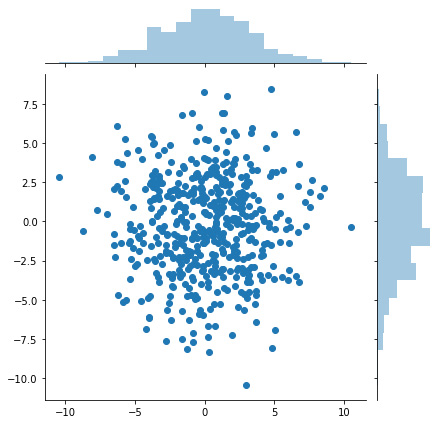Figure 1.7 – Joint distribution plot of a multi-dimensional continuous policy

6. Now, we are ready to implement the continuous policy class:
```class ContinuousPolicy(object):
def __init__(self, action_dim):
self.action_dim = action_dim
def sample(self, mu, var):
self.distribution = \
tfp.distributions.Normal(loc=mu, scale=sigma)
return self.distribution.sample(1)
def get_action(self, mu, var):
action = self.sample(mu, var)
return action```
7. As a next step, let's implement a multi-dimensional continuous policy class:
```import tensorflow_probability as tfp
import numpy as np
class ContinuousMultiDimensionalPolicy(object):
def __init__(self, num_actions):
self.action_dim = num_actions
def sample(self, mu, covariance_diag):
self.distribution = tfp.distributions.\
MultivariateNormalDiag(loc=mu,
scale_diag=covariance_diag)
return self.distribution.sample(1)
def get_action(self, mu, covariance_diag):
action = self.sample(mu, covariance_diag)
return action```
8. Let's now implement a function to evaluate an agent in an environment with a continuous action space to assess episodic performance:
```def evaluate(agent, env, render=True):
obs, episode_reward, done, step_num = env.reset(),
0.0, False, 0
while not done:
action = agent.get_action(obs)
obs, reward, done, info = env.step(action)
episode_reward += reward
step_num += 1
if render:
env.render()
return step_num, episode_reward, done, info```
9. We are now ready to test the agent in a continuous action environment:
```from neural_agent import Brain
import gym
env = gym.make("MountainCarContinuous-v0")Implementing a Neural-network Brain class using TensorFlow 2.x.
class Brain(keras.Model):
def __init__(self, action_dim=5,
input_shape=(1, 8 * 8)):
"""Initialize the Agent's Brain model
Args:
action_dim (int): Number of actions
"""
super(Brain, self).__init__()
self.dense1 = layers.Dense(32,
input_shape=input_shape, activation="relu")
self.logits = layers.Dense(action_dim)
def call(self, inputs):
x = tf.convert_to_tensor(inputs)
if len(x.shape) >= 2 and x.shape != 1:
x = tf.reshape(x, (1, -1))
return self.logits(self.dense1(x))
def process(self, observations):
# Process batch observations using `call(inputs)`
# behind-the-scenes
action_logits = \
self.predict_on_batch(observations)
return action_logits```
10. Let's implement a simple agent class that utilizes the `ContinuousPolicy` object to act in continuous action space environments:
```class Agent(object):
def __init__(self, action_dim=5,
input_dim=(1, 8 * 8)):
self.brain = Brain(action_dim, input_dim)
self.policy = ContinuousPolicy(action_dim)
def get_action(self, obs):
action_logits = self.brain.process(obs)
action = self.policy.get_action(*np.\
squeeze(action_logits, 0))
return action```
11. As a final step, we will test the performance of the agent in a continuous action space environment:
```from neural_agent import Brain
import gym
env = gym.make("MountainCarContinuous-v0")
action_dim = 2 * env.action_space.shape
# 2 values (mu & sigma) for one action dim
agent = Agent(action_dim, env.observation_space.shape)
steps, reward, done, info = evaluate(agent, env)
print(f"steps:{steps} reward:{reward} done:{done} info:{info}")
env.close()```

The preceding script will call the `MountainCarContinuous` environment, render it to the screen, and show how the agent is performing in this continuous action space environment: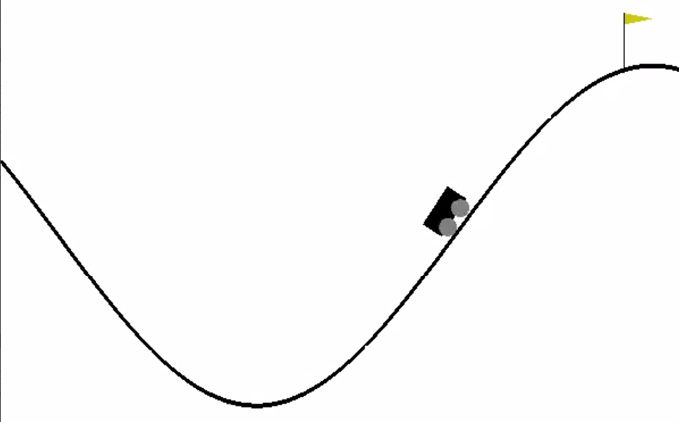Figure 1.8 – A screenshot of the agent in the MountainCarContinuous-v0 environment

Next, let's explore how it works.

## How it works…

We implemented a continuous-valued policy for RL agents using a Gaussian distribution. Gaussian distribution, which is also known as normal distribution, is the most widely used distribution for real numbers. It is represented using two parameters, µ and σ. We generated continuous-valued actions from such a policy by sampling from the distribution, based on the probability density that is given by the following equation: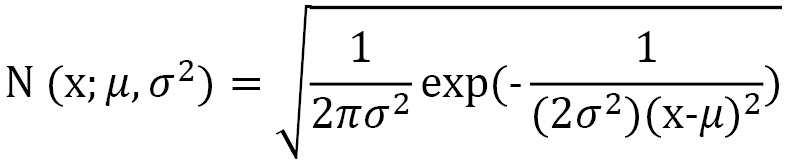The multivariate normal distribution extends the normal distribution to multiple variables. We used this distribution to generate multi-dimensional continuous policies.

# Working with OpenAI Gym for RL training environments

This recipe provides a quick run-through for getting up and running with OpenAI Gym environments. The Gym environment and the interface provide a platform for training RL agents and is the most widely used and accepted RL environment interface.

We will be needing the full installation of OpenAI Gym to be able to use the available environments. Please follow the Gym installation steps listed at https://github.com/openai/gym#id5.

As a minimum, you should execute the following command:

`pip install gym[atari]`

## How to do it…

Let's start by picking an environment and exploring the Gym interface. You may already be familiar with the basic function calls to create a Gym environment from the previous recipes.

Your steps should be formatted like so:

1. Let's first explore the list of environments in Gym:
```#!/usr/bin/env python
from gym import envs
env_names = [spec.id for spec in envs.registry.all()]
for name in sorted(env_names):
print(name)```
2. This script will print the names of all the environments available through your Gym installation, sorted alphabetically. You can run this script using the following command to see the names of the environments that are installed and available in your system. You should see a long list of environments listed. The first few are shown in the following screenshot for your reference: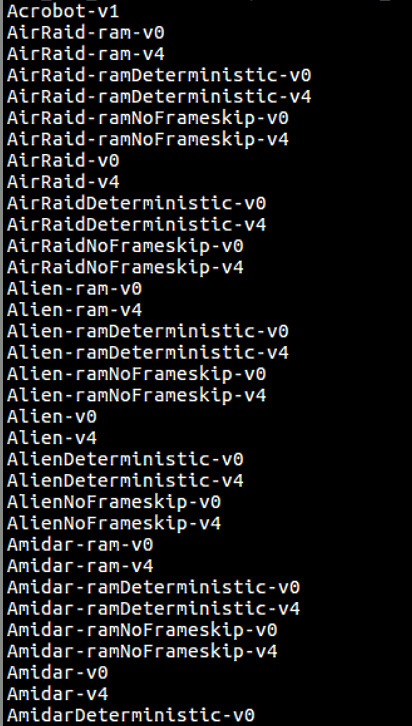Figure 1.9 – List of environments available using the openai-gym package

Let's now see how we can run one of the Gym environments.

3. The following script will let you explore any of the available Gym environments:
```#!/usr/bin/env python
import gym
import sys
def run_gym_env(argv):
env = gym.make(argv) # Name of the environment
# supplied as 1st argument
env.reset()
for _ in range(int(argv)):
env.render()
env.step(env.action_space.sample())
env.close()
if __name__ == "__main__":
run_gym_env(sys.argv)```
4. You can save the preceding script to `run_gym_env.py` and run the script like this:
`(tf2rl-cookbook) praveen@g5: ~/tf2rl-cookbook/ch1/src\$python run_gym_env.py Alien-v4 1000`

The script will render the `Alien-v4` environment, which should look like the following screenshot: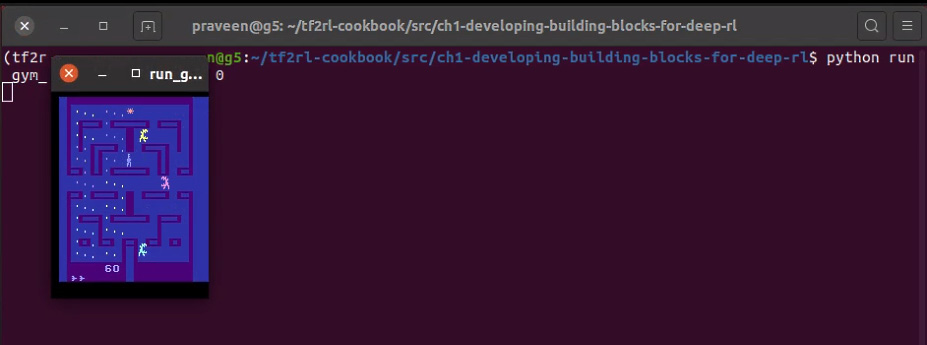Figure 1.10 – Sample output of run_gym_env.py with Alien-v4 1000 as the arguments

Tip

You can change `Alien-v4` to any of the available Gym environments listed in the previous step.

## How it works…

A summary of how the Gym environments work is presented in the following table: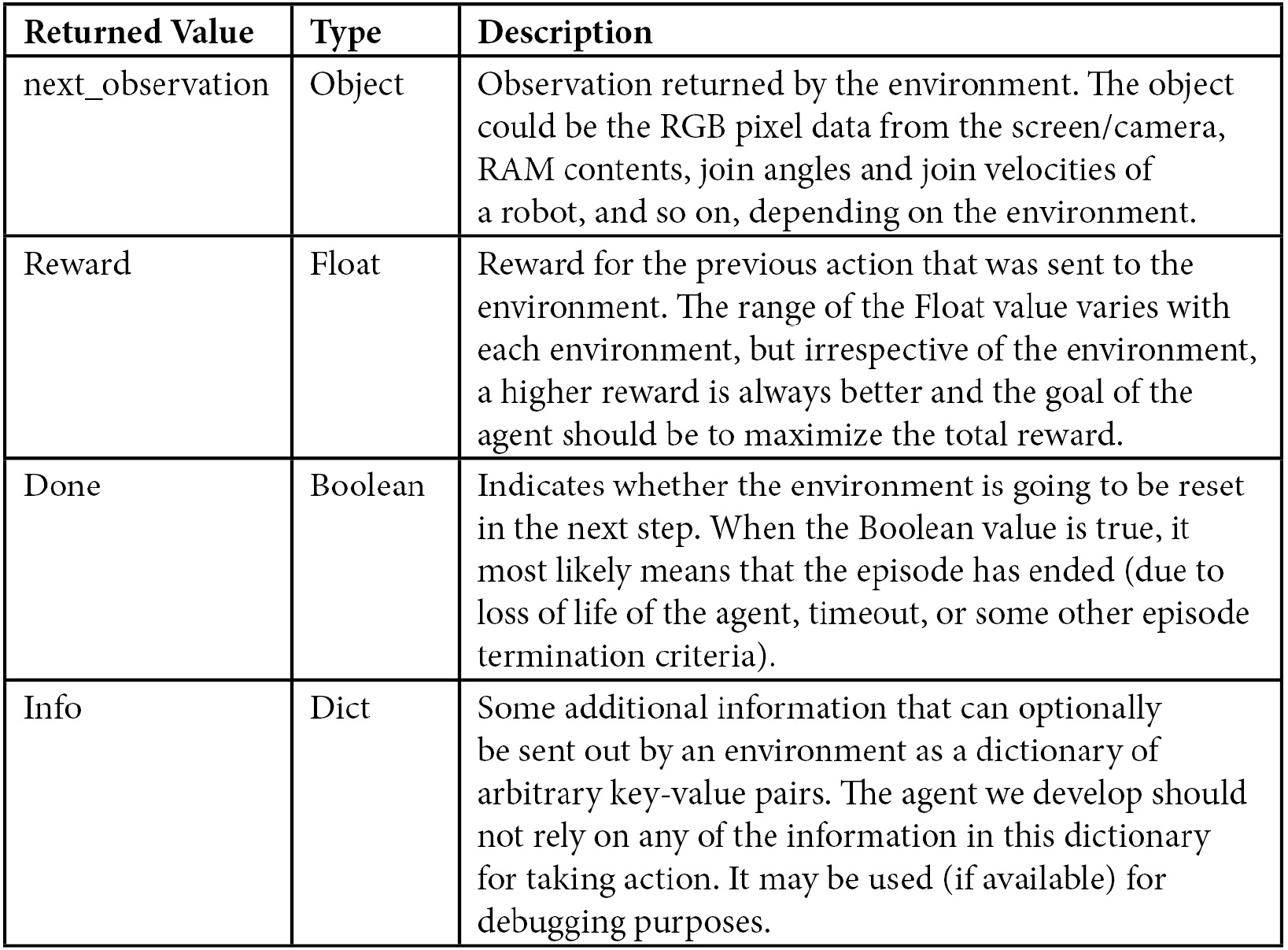Table 1.1 – Summary of the Gym environment interface

# Building a neural agent

This recipe will guide you through the steps to build a complete agent and the agent-environment interaction loop, which is the main building block for any RL application. When you complete the recipe, you will have an executable script where a simple agent tries to act in a Gridworld environment. A glimpse of what the agent you build will likely be doing is shown in the following screenshot: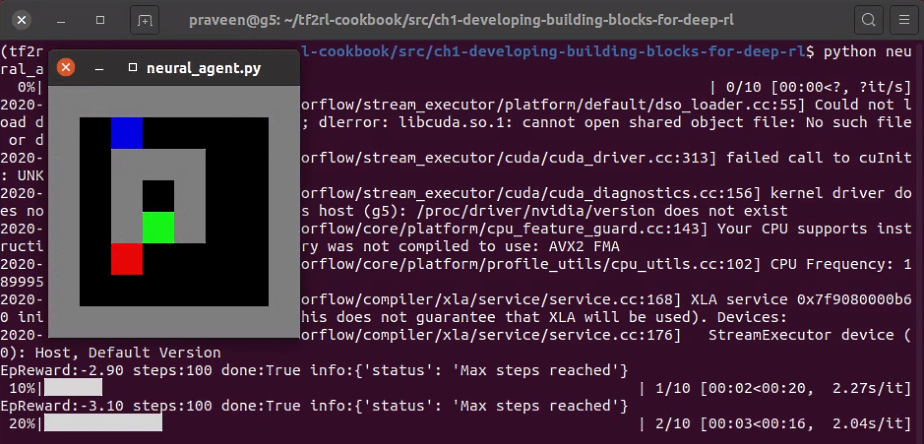Figure 1.11 – Screenshot of output from the neural_agent.py script

Let's get started by activating the `tf2rl-cookbook` Conda Python environment and running the following code to install and import the necessary Python modules:

```pip install tensorflow gym tqdm  # Run this line in a terminal
import tensorflow as tf
from tensorflow import keras
from tensorflow.keras import layers
import gym
import envs
from tqdm import tqdm```

## How to do it…

We will start by implementing a Brain class powered by a neural network implemented using TensorFlow 2.x:

1. Let's first initialize a neural brain model using TensorFlow 2.x and the Keras functional API:
```class Brain(keras.Model):
def __init__(self, action_dim=5,
input_shape=(1, 8 * 8)):
"""Initialize the Agent's Brain model
Args:
action_dim (int): Number of actions
"""
super(Brain, self).__init__()
self.dense1 = layers.Dense(32, input_shape= \
input_shape, activation="relu")
self.logits = layers.Dense(action_dim)```
2. Next, we implement the Brain class's `call(…)` method:
```def call(self, inputs):
x = tf.convert_to_tensor(inputs)
if len(x.shape) >= 2 and x.shape != 1:
x = tf.reshape(x, (1, -1))
return self.logits(self.dense1(x))```
3. Now we need to implement the Brain class's `process()` method to conveniently perform predictions on a batch of inputs/observations:
```def process(self, observations):
# Process batch observations using `call(inputs)`
# behind-the-scenes
action_logits = \
self.predict_on_batch(observations)
return action_logits```
4. Let's now implement the init function of the agent class:
```class Agent(object):
def __init__(self, action_dim=5,
input_shape=(1, 8 * 8)):
"""Agent with a neural-network brain powered
policy
Args:
brain (keras.Model): Neural Network based
model
"""
self.brain = Brain(action_dim, input_shape)
self.policy = self.policy_mlp```
5. Now let's define a simple policy function for the agent:
```def policy_mlp(self, observations):
observations = observations.reshape(1, -1)
# action_logits = self.brain(observations)
action_logits = self.brain.process(observations)
action = tf.random.categorical(tf.math.\
log(action_logits), num_samples=1)
return tf.squeeze(action, axis=1)```
6. After that, let's implement a convenient `get_action` method for the agent:
```def get_action(self, observations):
return self.policy(observations)```
7. Let's now create a placeholder function for `learn()` that will be implemented as part of RL algorithm implementation in future recipes:
```def learn(self, samples):
raise NotImplementedError```

This completes our basic agent implementation with the necessary ingredients!

8. Let's now evaluate the agent in a given environment for one episode:
```def evaluate(agent, env, render=True):
obs, episode_reward, done, step_num = env.reset(),
0.0, False, 0
while not done:
action = agent.get_action(obs)
obs, reward, done, info = env.step(action)
episode_reward += reward
step_num += 1
if render:
env.render()
return step_num, episode_reward, done, info```
9. Finally, let's implement the main function:
```if __name__ == "__main__":
env = gym.make("Gridworld-v0")
agent = Agent(env.action_space.n,
env.observation_space.shape)
for episode in tqdm(range(10)):
steps, episode_reward, done, info = \
evaluate(agent, env)
print(f"EpReward:{episode_reward:.2f}\
steps:{steps} done:{done} info:{info}")
env.close()```
10. Execute the script as follows:
`python neural_agent.py`

You should see the Gridworld environment GUI pop up. This will show you what the agent is doing in the environment, and it will look like the following screenshot: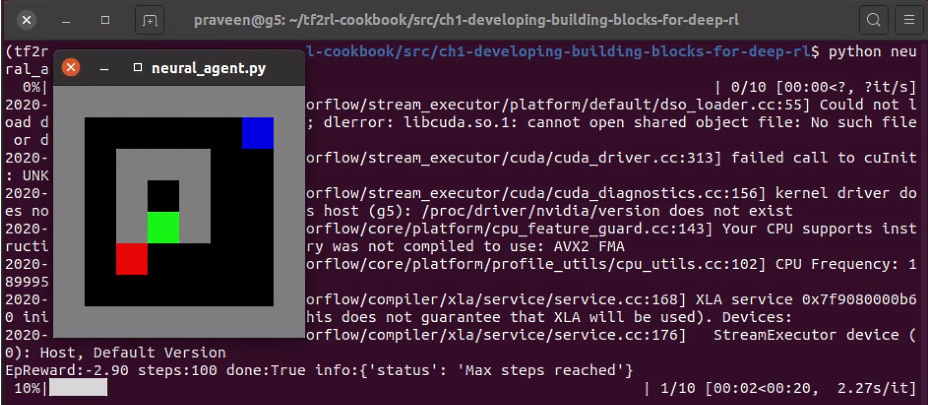Figure 1.12 – A screenshot of the neural agent acting in the Gridworld environment

This provides a simple, yet complete, recipe to build an agent and the agent-environment interaction loop. All that is left is to add the RL algorithm of your choice to the `learn()` method and the agent will start acting intelligently!

## How it works…

This recipe puts together the necessary ingredients to build a complete agent-environment system. The `Brain` class implements the neural network that serves as the processing unit of the agent, and the agent class utilizes the `Brain` class and a simple policy that chooses an action based on the output of the brain after processing the observations received from the environment.

We implemented the `Brain` class as a subclass of the `keras.Model` class, which allows us to define a custom neural network-based model for the agent's brain. The `__init__` method initializes the `Brain` model and defines the necessary layers using the TensorFlow 2.x Keras functional API. In this `Brain` model, we are creating two dense (also known as fully-connected) layers to build our starter neural network. In addition to the `__init__` method, the `call(…)` method is also a mandatory method that needs to be implemented by child classes inheriting from the `keras.Model` class. The `call(…) `method first converts the inputs to a TensorFlow 2.x tensor and then flattens the inputs to be of the shape `1 x total_number_of_elements` in the input tensor. For example, if the input data has a shape of 8 x 8 (8 rows and 8 columns), the data is first converted to a tensor and the shape is flattened to 1 x 8 * 8 = 1 x 64. The flattened inputs are then processed by the dense1 layer, which contains 32 neurons and a ReLU activation function. Finally, the logits layer processes the output from the previous layer and produces n number of outputs corresponding to the action dimension (n).

The `predict_on_batch(…)` method performs predictions on the batch of inputs given as the argument. This function (unlike the `predict()` function of Keras) assumes that the inputs (observations) provided as the argument are exactly one batch of inputs and thus feeds the batch to the network without any further splitting of the input data.

We then implemented the `Agent` class and, in the agent initialization function, we created an object instance of the Brain class by defining the following:

`self.brain = Brain(action_dim, input_shape)`

Here, `input_shape` is the shape of the input that is expected to be processed by the brain, and `action_dim` is the shape of the output expected from the brain. The agent's policy is defined to be a custom Multi-Layer Perceptron (MLP)-based policy based on the brain's neural network architecture. Note that we can reuse `DiscretePolicy` from the previous recipe to initialize the agent's policy as well.

The agent's policy function, `policy_mlp`, flattens the input observations and sends it for processing by the agent's brain to receive the `action_logits`, which are the unnormalized probabilities for the actions. The final action to be taken is obtained by using TensorFlow 2.x's `categorical` method from the random module, which samples a valid action from the given `action_logits` (unnormalized probabilities).

Important note

If all of the observations supplied to the `predict_on_batch` function cannot be accommodated in the given amount of GPU memory or RAM (CPU), the operation can cause a GPU Out Of Memory (OOM) error.

The main function that gets launched – if the `neural_agent.py` script is run directly – creates an instance of the Gridworld-v0 environment, initializes an agent using the action and observation space of this environment, and starts evaluating the agent for 10 episodes.

# Building a neural evolutionary agent

Evolutionary methods are based on black-box optimization and are also known as gradient-free methods since no gradient computation is involved. This recipe will walk you through the steps for implementing a simple, approximate cross-entropy-based neural evolutionary agent using TensorFlow 2.x.

Activate the `tf2rl-cookbook` Python environment and import the following packages necessary to run this recipe:

```from collections import namedtuple
import gym
import matplotlib.pyplot as plt
import numpy as np
import tensorflow as tf
from tensorflow import keras
from tensorflow.keras import layers
from tqdm import tqdm
import envs```

With the packages installed, we are ready to begin.

## How to do it…

Let's put together all that we have learned in this chapter to build a neural agent that improves its policy to navigate the Gridworld environment using an evolutionary process:

1. Let's start by importing the basic neural agent and the Brain class from `neural_agent.py`:
```from neural_agent import Agent, Brain
from envs.gridworld import GridworldEnv```
2. Next, let's implement a method to roll out the agent in a given environment for one episode and return `obs_batch`, `actions_batch`, and `episode_reward`:
```def rollout(agent, env, render=False):
obs, episode_reward, done, step_num = env.reset(),
0.0, False, 0
observations, actions = [], []
episode_reward = 0.0
while not done:
action = agent.get_action(obs)
next_obs, reward, done, info = env.step(action)
# Save experience
observations.append(np.array(obs).reshape(1, -1))  	        # Convert to numpy & reshape (8, 8) to (1, 64)
actions.append(action)
episode_reward += reward

obs = next_obs
step_num += 1
if render:
env.render()
env.close()
return observations, actions, episode_reward```
3. Let's now test the trajectory rollout method:
```env = GridworldEnv()
# input_shape = (env.observation_space.shape * \
env.observation_space.shape, )
brain = Brain(env.action_space.n)
agent = Agent(brain)
obs_batch, actions_batch, episode_reward = rollout(agent,
env)```
4. Now, it's time for us to verify that the experience data generated using the rollouts is coherent:
`assert len(obs_batch) == len(actions_batch)`
5. Let's now roll out multiple complete trajectories to collect experience data:
```# Trajectory: (obs_batch, actions_batch, episode_reward)
# Rollout 100 episodes; Maximum possible steps = 100 * 100 = 10e4
trajectories = [rollout(agent, env, render=True) \
for _ in tqdm(range(100))]```
6. We can then visualize the reward distribution from a sample of experience data. Let's also plot a red vertical line at the 50th percentile of the episode reward values in the collected experience data:
```from tqdm.auto import tqdm
import matplotlib.pyplot as plt
%matplotlib inline
sample_ep_rewards = [rollout(agent, env)[-1] for _ in \
tqdm(range(100))]
plt.hist(sample_ep_rewards, bins=10, histtype="bar");```

Running this code will generate a plot like the one shown in the following diagram: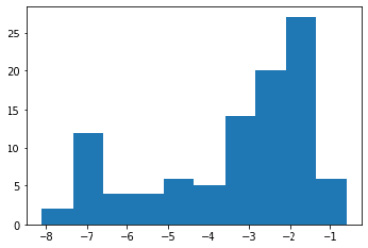Figure 1.13 – Histogram plot of the episode reward values

7. Let's now create a container for storing trajectories:
```from collections import namedtuple
Trajectory = namedtuple("Trajectory", ["obs", "actions",
"reward"])
# Example for understanding the operations:
print(Trajectory(*(1, 2, 3)))
# Explanation: `*` unpacks the tuples into individual
# values
Trajectory(*(1, 2, 3)) == Trajectory(1, 2, 3)
# The rollout(...) function returns a tuple of 3 values:
# (obs, actions, rewards)
# The Trajectory namedtuple can be used to collect
# and store mini batch of experience to train the neuro
# evolution agent
trajectories = [Trajectory(*rollout(agent, env)) \
for _ in range(2)]```
8. Now it's time to choose elite experiences for the evolution process:
```def gather_elite_xp(trajectories, elitism_criterion):
"""Gather elite trajectories from the batch of
trajectories
Args:
batch_trajectories (List): List of episode \
trajectories containing experiences (obs,
actions,episode_reward)
Returns:
elite_batch_obs
elite_batch_actions
elite_reard_threshold

"""
batch_obs, batch_actions,
batch_rewards = zip(*trajectories)
reward_threshold = np.percentile(batch_rewards,
elitism_criterion)
indices = [index for index, value in enumerate(
batch_rewards) if value >= reward_threshold]

elite_batch_obs = [batch_obs[i] for i in indices]
elite_batch_actions = [batch_actions[i] for i in \
indices]
unpacked_elite_batch_obs = [item for items in \
elite_batch_obs for item in items]
unpacked_elite_batch_actions = [item for items in \
elite_batch_actions for item in items]
return np.array(unpacked_elite_batch_obs), \
np.array(unpacked_elite_batch_actions), \
reward_threshold```
9. Let's now test the elite experience gathering routine:
`elite_obs, elite_actions, reward_threshold = gather_elite_xp(trajectories, elitism_criterion=75)`
10. Let's now look at implementing a helper method to convert discrete action indices to one-hot encoded vectors or probability distribution over actions:
```def gen_action_distribution(action_index, action_dim=5):
action_distribution = np.zeros(action_dim).\
astype(type(action_index))
action_distribution[action_index] = 1
action_distribution = \
np.expand_dims(action_distribution, 0)
return action_distribution```
11. It's now time to test the action distribution generation function:
`elite_action_distributions = np.array([gen_action_distribution(a.item()) for a in elite_actions])`
12. Now, let's create and compile the neural network brain with TensorFlow 2.x using the Keras functional API:
```brain = Brain(env.action_space.n)
13. You can now test the brain training loop as follows:
```elite_obs, elite_action_distributions = elite_obs.astype("float16"), elite_action_distributions.astype("float16")
brain.fit(elite_obs, elite_action_distributions, batch_size=128, epochs=1);```

This should produce the following output:

`1/1 [==============================] - 0s 960us/step - loss: 0.8060 - accuracy: 0.4900`

Note

The numbers may vary.

14. The next big step is to implement an agent class that can be initialized with a brain to act in an environment:
```class Agent(object):
def __init__(self, brain):
"""Agent with a neural-network brain powered
policy
Args:
brain (keras.Model): Neural Network based \
model
"""
self.brain = brain
self.policy = self.policy_mlp
def policy_mlp(self, observations):
observations = observations.reshape(1, -1)
action_logits = self.brain.process(observations)
action = tf.random.categorical(
tf.math.log(action_logits), num_samples=1)
return tf.squeeze(action, axis=1)
def get_action(self, observations):
return self.policy(observations)```
15. Next, we will implement a helper function to evaluate the agent in a given environment:
```def evaluate(agent, env, render=True):
obs, episode_reward, done, step_num = env.reset(),
0.0, False, 0
while not done:
action = agent.get_action(obs)
obs, reward, done, info = env.step(action)
episode_reward += reward
step_num += 1
if render:
env.render()
return step_num, episode_reward, done, info```
16. Let's now test the agent evaluation loop:
```env = GridworldEnv()
agent = Agent(brain)
for episode in tqdm(range(10)):
steps, episode_reward, done, info = evaluate(agent,
env)
env.close()```
17. As a next step, let's define the parameters for the training loop:
```total_trajectory_rollouts = 70
elitism_criterion = 70  # percentile
num_epochs = 200
mean_rewards = []
elite_reward_thresholds = []```
18. Let's now create the `environment`, `brain`, and `agent` objects:
```env = GridworldEnv()
input_shape = (env.observation_space.shape * \
env.observation_space.shape, )
brain = Brain(env.action_space.n)
brain.compile(loss="categorical_crossentropy",
agent = Agent(brain)
for i in tqdm(range(num_epochs)):
trajectories = [Trajectory(*rollout(agent, env)) \
for _ in range(total_trajectory_rollouts)]
_, _, batch_rewards = zip(*trajectories)
elite_obs, elite_actions, elite_threshold = \
gather_elite_xp(trajectories,
elitism_criterion=elitism_criterion)
elite_action_distributions = \
np.array([gen_action_distribution(a.item()) \
for a in elite_actions])
elite_obs, elite_action_distributions = \
elite_obs.astype("float16"),
elite_action_distributions.astype("float16")
brain.fit(elite_obs, elite_action_distributions,
batch_size=128, epochs=3, verbose=0);
mean_rewards.append(np.mean(batch_rewards))
elite_reward_thresholds.append(elite_threshold)
print(f"Episode#:{i + 1} elite-reward-\
threshold:{elite_reward_thresholds[-1]:.2f} \
reward:{mean_rewards[-1]:.2f} ")
plt.plot(mean_rewards, 'r', label="mean_reward")
plt.plot(elite_reward_thresholds, 'g',
label="elites_reward_threshold")
plt.legend()
plt.grid()
plt.show()```

This will generate a plot like the one shown in the following diagram:

Important note

The episode rewards will vary and the plots may look different.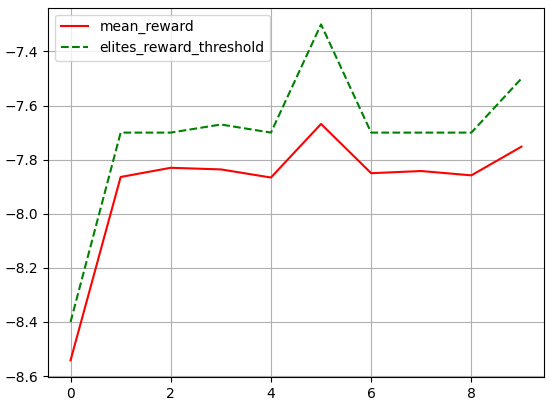Figure 1.14 – Plot of the mean reward (solid, red) and reward threshold for elites (dotted, green)

The solid line in the plot is the mean reward obtained by the neural evolutionary agent, and the dotted line shows the reward threshold used for determining the elites.

## How it works…

On every iteration, the evolutionary process rolls out or collects a bunch of trajectories to build up the experience data using the current set of neural weights in the agent's brain. An elite selection process is then employed that picks the top k percentile (elitism criterion) trajectories/experiences based on the episode reward obtained in that trajectory. This shortlisted experience data is then used to update the agent's brain model. The process repeats for a preset number of iterations allowing the agent's brain model to improve and collect more rewards.